# Class 12 Nuclear Physics

4575

Physics Modern Physics Level: Misc Level

Nearly all the mass of an atom is concentrated in its nucleus. Where is its charge located?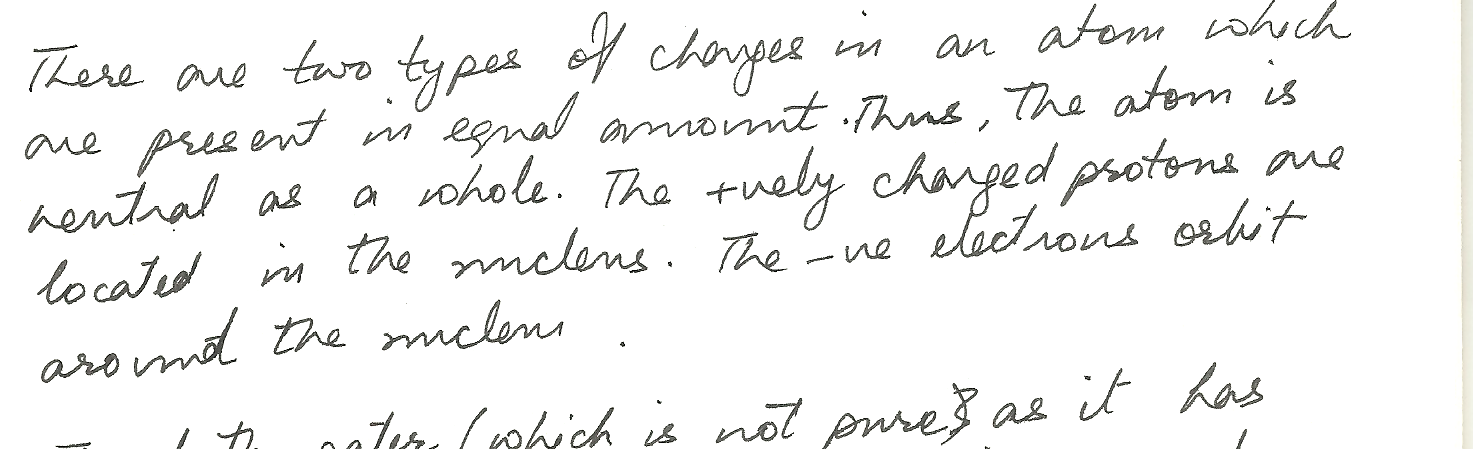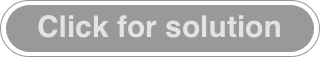4482

Physics Modern Physics Level: Misc Level

How many fissions take place per second in a 200 MW reactor? Assume 200 MeV is released per fission.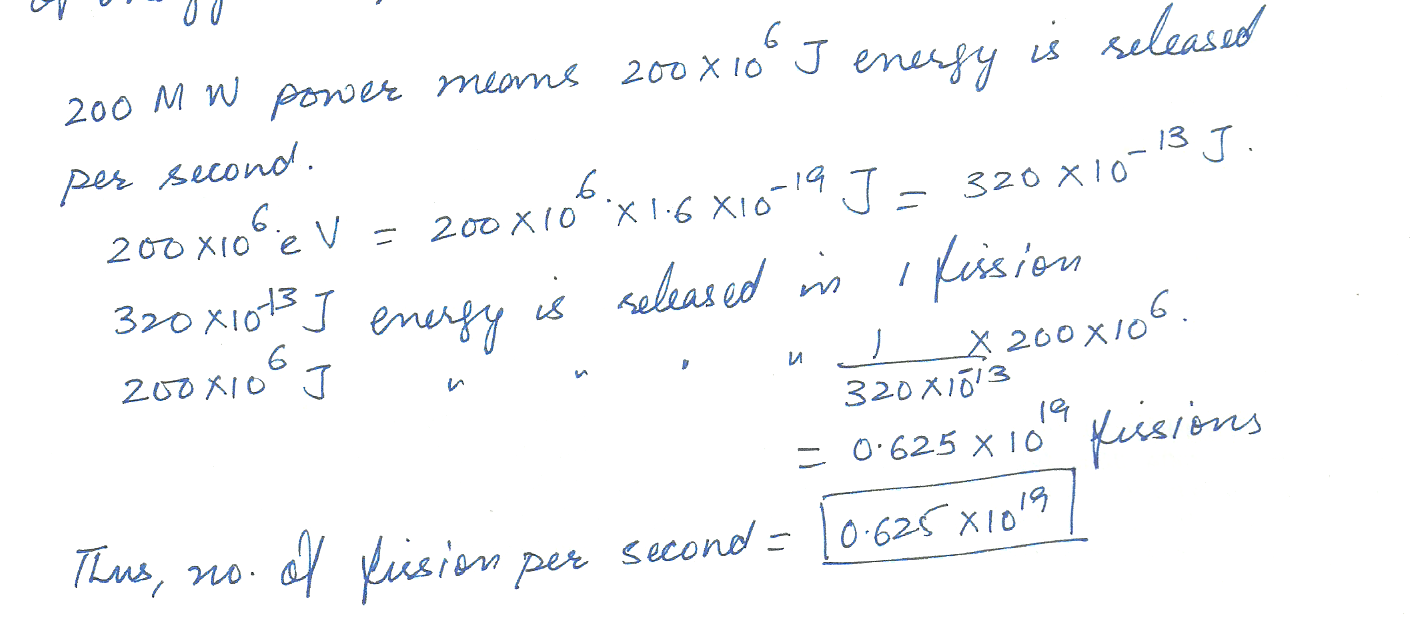4480

Physics Modern Physics Level: Misc Level

What fraction of a sample is left after exactly 5 half - lives?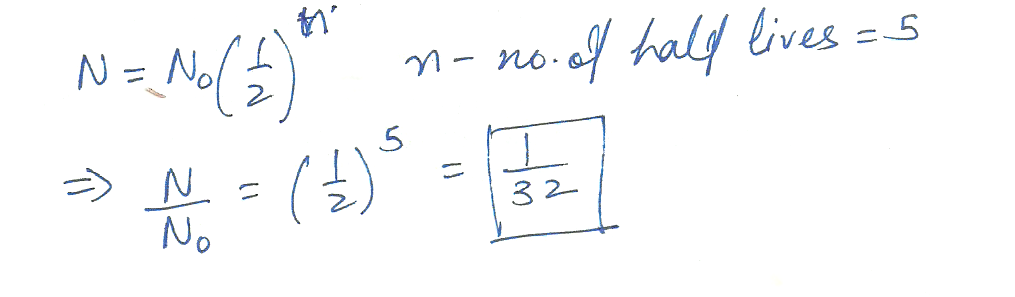4478

Physics Modern Physics Level: Misc Level

What is the approximate radius of an alpha particle ( 4 2 He)?4019

Physics Modern Physics Level: University

Mass Energy Relationship
1 kg of gasoline when burned in oxygen will give off about 4 x 10^7 joules of heat. According to Einstein any mass has an equivalent energy of E = mc^2. How  many  kgs of gasoline would have to burn ( with oxygen) to make the same amount of energy that Einsteins theory predicts that a single kg is equivalent to?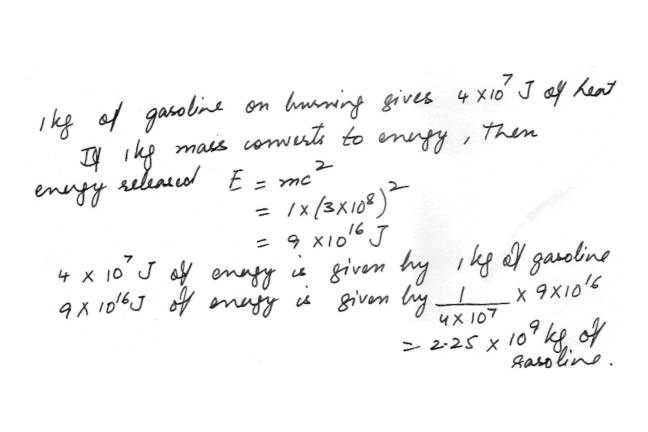3944

Physics Modern Physics Level: University

Assume initially that the Boron atom created in the rarioactive decay of 4^11 Be is electrically neutral. How many protons how many neutrons and how many electrons does the Boron atom contain?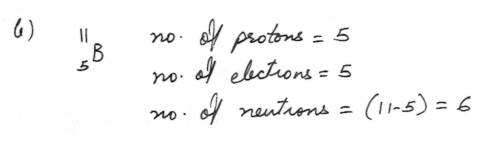3943

Physics Modern Physics Level: University

One isotope of Beryllium 4^11 Be undergoes beta- minus decay with a half-life of around 14 seconds write an equation for the beta-minus decay of a4^11 Be atom showing the symbols for the Boron isotope that is produced and for the other particles that are emitted.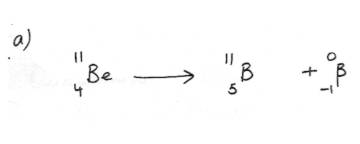3218

Physics Modern Physics Level: High School

Compare the energy released per kilogram of deuterium for D-T fusion to the energy released per kilogram of 235U for 235U fission.

Note: A gram of deuterium contains 3.01 x 1023 atoms.

3078

Physics Modern Physics Level: High School

How many radioactive atoms are present in a sample that has an activity of 0.5 microCi and a half-life of 10 years ? (1 curie = 3.7 x 1010 decays/ s )

a) 9.5 x E22 atoms

b) 8.4 x E12 atoms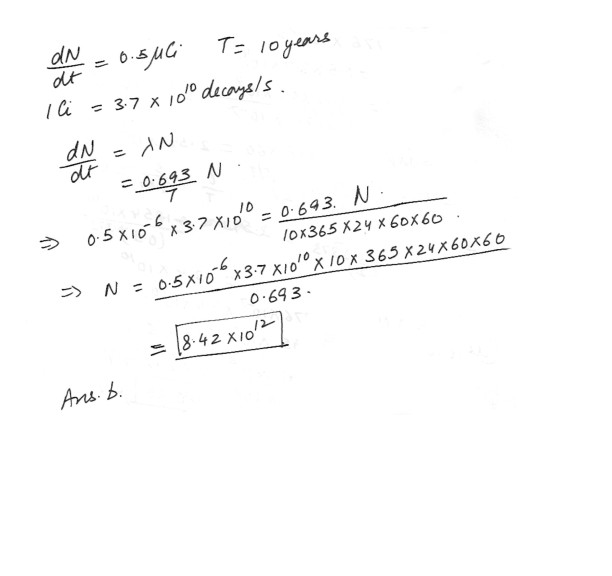3077

Physics Modern Physics Level: High School

44 g of petrified wood was found in a petrified forest . A sample showed a 14 C activity of 100 decays / minute . How long has the tree been dead (in years) ? (The half-time of carbon -14 is 5730 years and freshly cut wood contains 6.5 x 1010 atoms of 14 C per gram) .

a) 32,300

b) 15,600

c) 8,500

d) 4,700

e) 2,400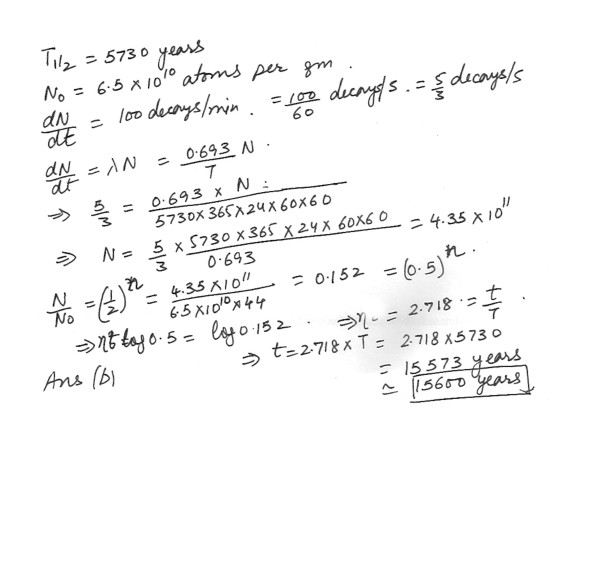Displaying 1-10 of 23 results.